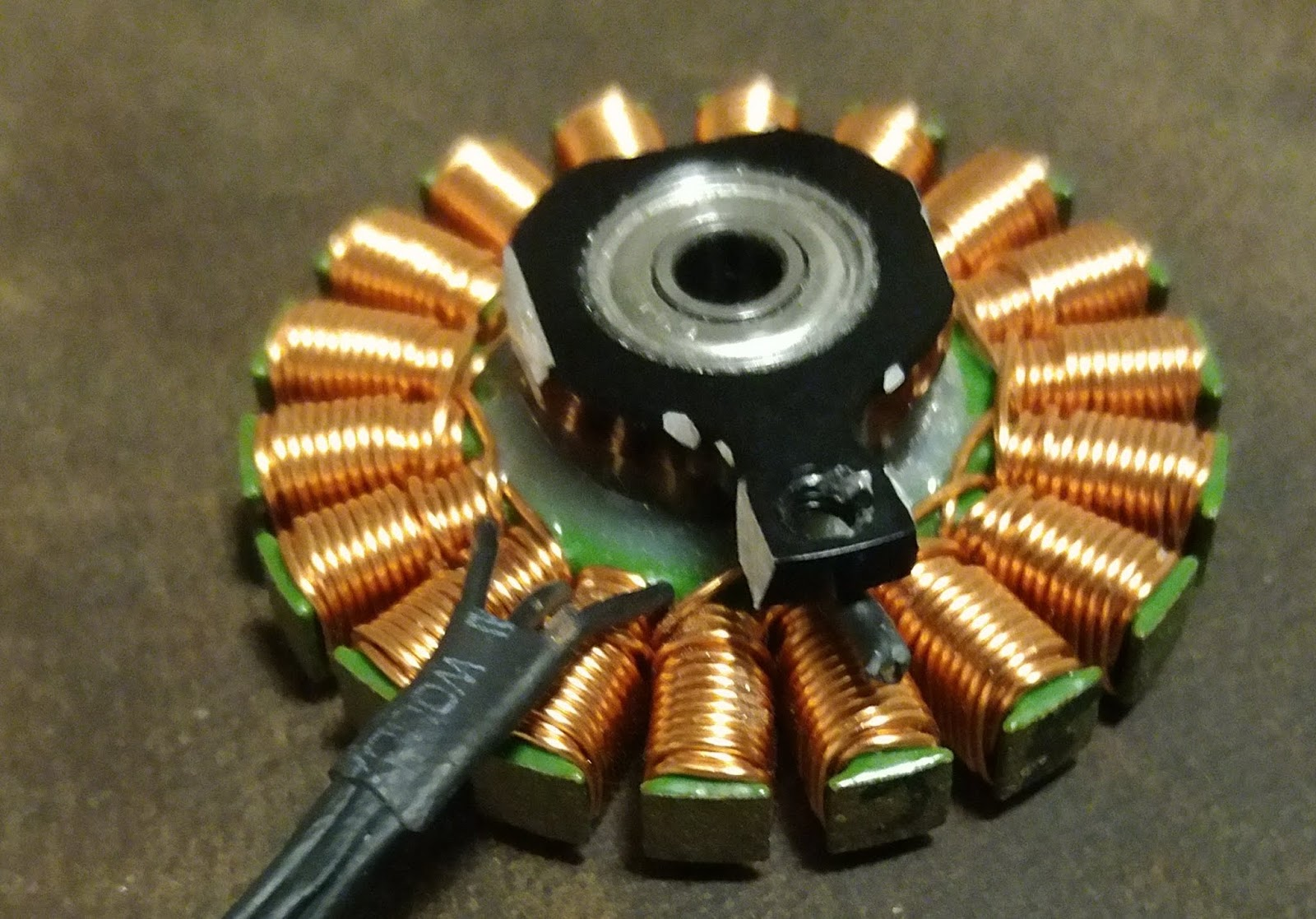# T-Motor 4004 - 300kvThis is the bottom view of the stater. 2 layers of winding per arm. The top layer has either 10 wire crossings or 9 crossings. Node 15 has 10 crossings and node 3 has 9 crossings, for example. The 9 crossings means 10 turns because the 10th turn takes the wire on to the next arm winding. When the top layer has 10 crossings, the lower layer has 14 turns for a total of 10+14+1=5 turns; when the top layer has 9 crossing, the lower layer has 15 turns for a total of 9+15+1=25 turns. When the lower layer has 15 turns, the wire looks squizzed near the arm's flared end. For example, node 3 has the lower layer's wire slight overflow while node 15 has the wire flush and fit in the arm.

1. wire C cut at the Y termination point.  2. wire C unwinds counter-clockwise on node 18.  3. wire c unwinds 10 full turns.

There are 14 wires crossing this bottom side of the stater arm. This is the only node that has 14 wires crossing the arm after unwinding top layer 10 turns. This is because the last node has the wire cross the arm to the WYE Y termination point, making it took like 11 turns on the top layer of the winding.  Regular nodes do not have this extra crossing. Factory worker was confused and counted the extra crossing as a turn.

## Winding

The 22 AWG wires are too thick and get into neighbor arms.

The wire length for 19 turns with the 22 AWG is 33.5cm.

The first 11 turns, when diameter is 24 AWG , 33.5 x 11 / 19 x (4+1)/(4+1.3) = 18.4 cm. Remaining 9 turn, when 24 Awg is used, should only need 33.5 x 9 / 19 x (4+2)/(4+2.6) = 14.4 cm. Total 32.8cm per arm. Actual winding only needs 29.5cm with 24AWG because the turning diameter saves 10%. Each continuous wire needs to be 29.5 x 6 + 5 = 177cm + 5cm = 182cm. 4cm for wire lead and 1cm for  Y termination solder.

3 strands

4 strands